Science Journal of Chemistry
Volume 4, Issue 3, June 2016, Pages: 29-35

Molecular Dynamics Calculation on Composition B Adsorption Water

Guiyun Hang*, Wenli Yu, Tao Wang, Zhen Li

Department of Nuclear Engineering, Xi’an Research Institute of High-Tech, Xi’an, Shaanxi, China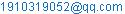(Guiyun Hang)

*Corresponding author

Guiyun Hang, Wenli Yu, Tao Wang, Zhen Li. Molecular Dynamics Calculation on Composition B Adsorption Water. Science Journal of Chemistry. Vol. 4, No. 3, 2016, pp. 29-35. doi: 10.11648/j.sjc.20160403.11

Received: May 25, 2016; Accepted: June 3, 2016; Published: June 21, 2016

Abstract: To research the adsorption mechanism of water on composition B crystal surfaces and the effect on mechanical properties and sensitivity of explosive, the crystal model of composition B was established by Material Studio (MS). The adsorption process was simulated and the mechanical properties, adsorption energy of different crystal surfaces, trigger bond length, interaction energy of trigger bond and cohesive energy density were got and compared. The results show that the (0 1 0) crystal surface has the best adsorption capacity, the mechanical properties decrease after adsorption and it is more obvious with the increasing of adsorbed gas number, which indicates that the mechanical properties of composition B become worse. The maximum trigger bond length decreases, while the interaction energy of trigger bond and cohesive energy density increase with the increasing of gas number, thus illustrating that the sensitivity of composition B decreases.

Keywords: Physical Chemistry, Composition B, Mechanical Properties, Sensitivity, Material Studio, Molecular Dynamics

1. Introduction

As one of the most important explosives, composition B consists of hexahydro-1,3,5-trinitro-1,3,5-triazine (RDX) and 2,4,6-trinitroto-luene (TNT) and it has notable merits such as high energy density, good safety and improved mechanical properties. So, composition B has been widely used in both military and industry areas for a long time [1-2].

During the storage process, influenced by heat and other factors, composition B may be decomposed and some correlated gases such as carbon dioxide, nitrogen, water and etc. would come into being. The gases would be adsorbed on the crystal surfaces of explosive and caused some chemical and physical reaction, which would change the mechanical properties and sensitivity of composition B further. Influenced by this, the performance and safety of the weapons will be affected. Therefore, it is of great significance to research the adsorption mechanism of gases and the effect on mechanical properties and sensitivity of explosive. This paper chose the water as the adsorbed gas and researched the influence of adsorption on mechanical properties and sensitivity of composition B.

To research the adsorption mechanism and simulate the adsorption process, the mainly used software is Material Studio (MS)  and molecular dynamics (MD) simulation is the commonly used method. Recently, a lot of researchers have used MS to conduct investigations on molecular structures, mechanical properties, sensitivity, stability, binding energy and detonation performance of energetic materials and high energy density explosives and a great deal of achievements have been got [4-13].

2. Calculation Model and Method

2.1. Molecular Model of RDX and TNT

Composition B consists of RDX and TNT, the molecular formula is C3H6O6N6, C7H5O6N3, respectively. The molecular models of RDX and TNT are shown in Fig. 1. (The grey represents carbon atom, the white represents hydrogen atom, the red represents oxygen atom and the blue represents nitrogen atom).

2.2. Crystal Model of Composition B

In composition B, the mass ratio of RDX and TNT is 60% and 40%, respectively, while the relative molecular weight of RDX is 222.1 and it is 227.1 for TNT. By calculation, we know that the total ratio of molecular number of RDX and TNT in composition B is 32. In this paper, the molecule number of TNT is 20, so the number of RDX is 30 and there are 1050 atoms in total.

Both of the 20 TNT molecules and 30 RDX molecules are put in a box with size 20 nm×20 nm×20 nm to ensure that all of the molecules have enough motion space and can contact with each other, then the size of the box is decreased along with the pressing of space and isothermal-isochoric ensemble (NVT) MD simulations are performed, at this time, the density of the explosive will be increased. The process will go on until the density of the explosive reaches the ideal value.

After the crystal has been established, MD simulations will be performed in Discover and Forcite modules to optimize the energy and structure of the model. Through optimization, the crystal parameters of composition B is a=2.295 nm, b=2.188 nm, c=2.185 nm, α=93.26°, β=92.02°, γ=92.88°. The crystal model of composition B is shown in Fig. 2.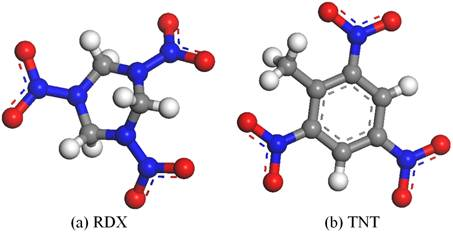Figure 1. Molecular models of RDX and TNT.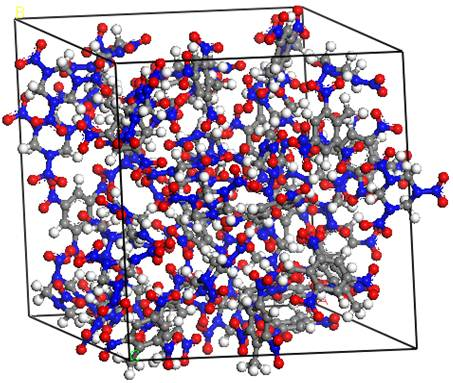Figure 2. Crystal model of composition B.

To simulate the adsorption process and investigate the differences among the properties of various crystalline surfaces, the crystal model was cleaved along three crystalline surfaces (1 0 0), (0 1 0), and (0 0 1), respectively to keep its integrality. Then the optimized water molecules are put into the three crystal surfaces above.

2.3. Mechanical Properties of Explosives

The relation between stress (σ) and strain (ε) of a material follows the generalized Hooke’ law [14-15] as follows: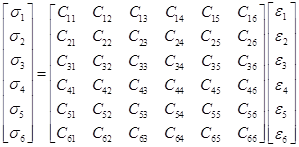(1)

Where Cij (i, j=1, 2, …, 6) is the matrix of elastic coefficients. The matrix is symmetric due to the existence of strain energy, therefore, 21 coefficients are required to describe the relationship between stress and strain for materials.

The mechanical properties for a material mainly conclude tensile modulus (E), bulk modulus (K), shear modulus (G), Poisson’s ratio (γ) and Cauchy pressure (C12C44). Tensile modulus, bulk modulus, and shear modulus can be used to assess the rigidity or stiffness of a material . Besides, mechanical properties can also be used to balance the ability for a material to resist elastic deformation. Meanwhile, the K value can also represent the rupture strength, that is, the greater the value of K is, the more energy will be required for a material to rupture. Cauchy pressure (C12C44) can be used as a criterion to evaluate the ductibility or brittleness of a material. As a rule, the value of (C12C44) for a ductile material is positive; on the contrary, it is negative for a brittle material. Besides, the more positive the (C12C44) value is, the more ductile the material is. All the mechanical properties can be obtained from the Lamé coefﬁcients (λ and μ) according to the following formulas: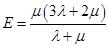(2)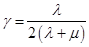(3)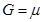(4)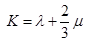(5)

2.4. Calculation Conditions

This paper mainly researches the adsorption mechanism of water on composition B crystal surfaces and the effect on mechanical properties and sensitivity of explosive. The number of adsorbed water is 5, 10, 15, 20, 25, 30, respectively. MD simulations are carried out in the NVT (constant number of particles, volume, and temperature) ensemble with the COMPASS force ﬁeld [17-19]. The temperature is 295 K and the step size is 1 fs and the total simulation time is 2×105 fs. The systems are first integrated for 1×105 time steps in all equilibration runs, which are necessary to attain the mechanical and thermal equilibrium, and then followed by the production runs of 1×105 time steps, during which data are collected for subsequent analysis. One frame is saved per 1000 steps, and totally 100 frames are saved to make analysis of static mechanical properties.

3. Results Analysis

3.1. Equilibrium of System

To analyze the static mechanical properties of composition B, the system must reach the equilibrium state. The equilibrium of system is ascertained by the equilibrium in temperature and energy simultaneously; that is to say, when the temperature and energy fluctuate in the range of 5~10%, the system is believed to have reached the equilibrium state.

Take the (0 1 0) crystal surface as an example, when the number of adsorbed water is 20, the temperature and energy curve versus time is shown in Fig. 3 and Fig. 4, respectively.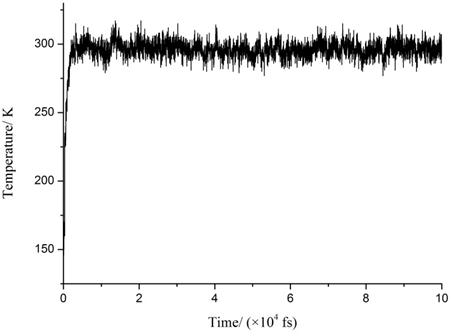Figure 3. Temperature curve versus time.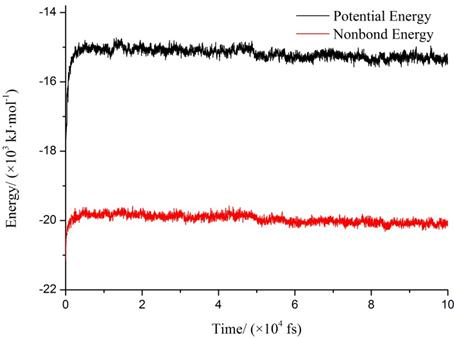Figure 4. Energy curve versus time.

What can be seen from Fig. 3 and Fig. 4 is that the temperature and energy increases at the beginning and then both of the temperature and energy curves fluctuate at a certain range and the system equilibrates in less than 5×104 fs. At last, the temperature fluctuates within ±15 K and the energy fluctuates with ±5~10%, which indicates that the system has reached the equilibrium state. For other conditions, the equilibrium state of the system is based on the two principles.

3.2. Adsorption Energy of Different Crystal Surfaces

The adsorption energy (Ea) is defined as follows: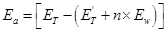(6)

Where Ea is the adsorption energy, ET is the equilibrium energy of the system before adsorption,is the equilibrium energy after adsorption, Ew refers the single molecule energy of water, n represents the number of adsorbed water. The value of adsorption energy is negative, and the more negative the value of adsorption energy (Ea) is, the better the adsorption capacity is.

The adsorption energy of different crystal surfaces is shown in Fig. 5.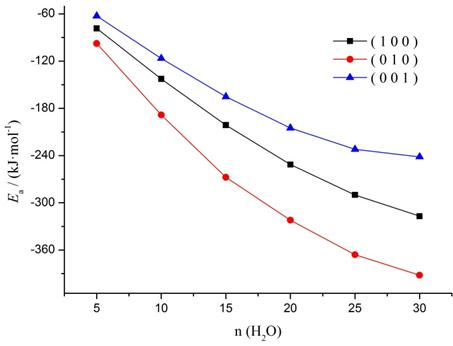Figure 5. Adsorption energy of different crystal surfaces.

It can be clearly drawn from Fig. 5 that the adsorption energy of the three crystal surfaces is different from each other. The adsorption energy value of (0 1 0) crystal surface is the most negative, thus indicating that (0 1 0) crystal surface has the best adsorption capacity, then comes to the (1 0 0) crystal surface and (0 0 1) crystal surface is the last. What’s more, we can also conclude that with the increasing of adsorbed gas number, the adsorption energy value decreases, which further implies that the adsorption capacity becomes better.

3.3. Mechanical Properties of Composition B Before and After Adsorption

When the system reaches the equilibrium state, calculations will be conducted under the Forcite modules in MS and we can get the mechanical properties of different crystal surfaces. For example, when the number of adsorbed water is 15, the elastic coefficients and mechanical properties (tensile modulus, Poisson’s ratio, bulk modulus, shear modulus and Cauchy pressure) of different crystal surfaces are shown in Table 1.

What can be clearly concluded from Table 1 is that after adsorption water, the mechanical properties of the three crystal surfaces have changed to some extent. Compared with the initial moduli, the mechanical properties (tensile modulus, bulk modulus, shear modulus and Cauchy pressure) decrease after adsorption, which illustrates that the rigidity of the explosive is reduced, i.e., the resistance to elastic deformation is decreased. Besides, these variations also suggest that the explosive will deform more easily when they are subjected to an external force, while Cauchy pressure decreasing means that the ductibility of the explosive becomes worse after adsorption. Therefore, adsorption water has a negative effect on the mechanical properties of composition B.

Table 1. Mechanical properties of different crystal surfaces before and after adsorptiona).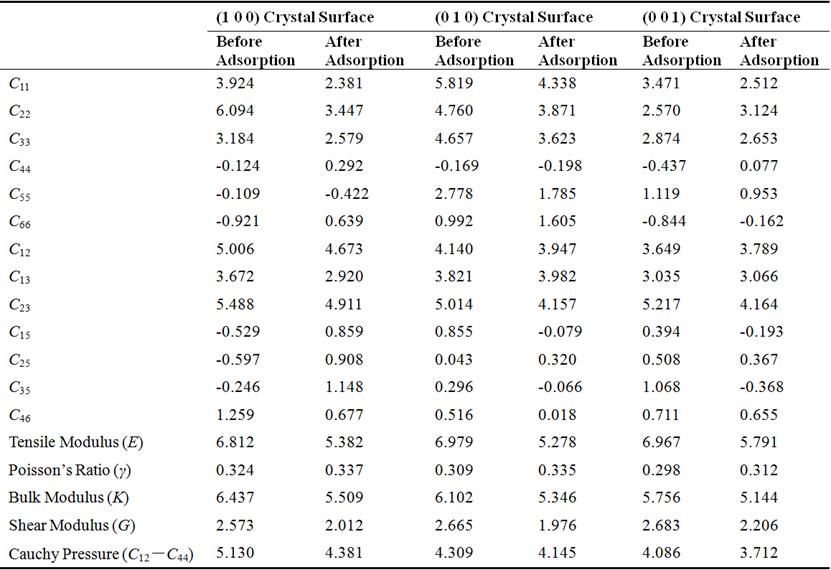a) Units for all the elastic coefficients and mechanical properties is GPa except for Poisson’s Ratio.

Table 2. Mechanical properties of (0 0 1) crystal surfaceb).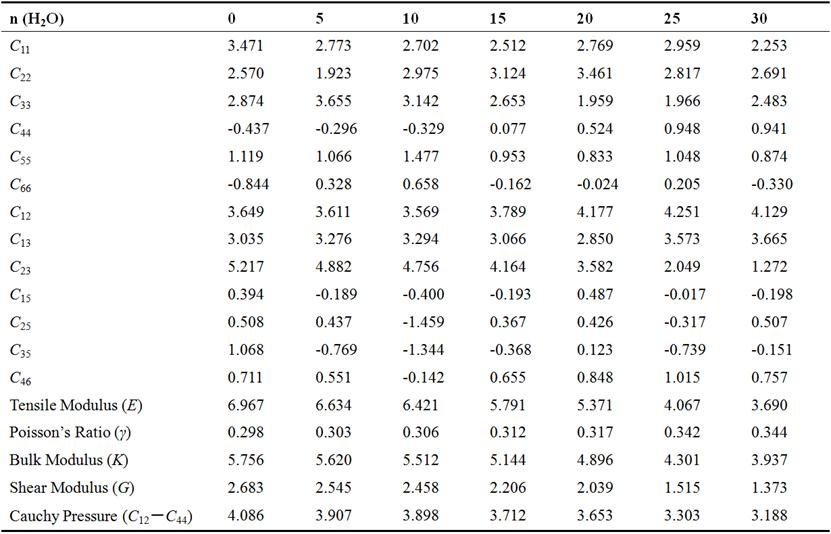b) Units for all the elastic coefficients and mechanical properties is GPa except for Poisson’s Ratio.

3.4. Effect of Adsorption Gas Number on Mechanical Properties

To research the effect of adsorption gas number on mechanical properties of explosive, take the (0 0 1) crystal surface as an example, Table 2 presents the mechanical properties of the explosive in different conditions and Fig. 6 gives the mechanical properties curves versus adsorption gas number.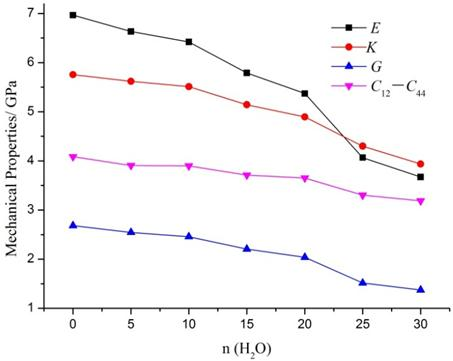Figure 6. Mechanical properties of (0 0 1) crystal surface.

What can be clearly seen from Table 2 and Fig. 6 is that the adsorption gas number has a direct effect on the mechanical properties of explosive. With the increasing of adsorption gas number, the mechanical properties (tensile modulus, bulk modulus, shear modulus and Cauchy pressure) decrease gradually and it is more obvious for the tensile modulus and shear modulus. Before adsorption, the tensile modulus is 6.967 GPa and the shear modulus is 2.683 GPa; after adsorption, the tensile modulus is 3.690 GPa and shear modulus is 1.373 GPa, the decreased extent of tensile modulus and shear modulus is 47.04%, 48.38%, respectively. While the decrease of bulk modulus is 31.60% and it is 21.98% for Cauchy pressure. The decreasing of mechanical properties implies that the rigidity and hardness of the explosive becomes worse, while the toughness and plastic property becomes better. Based on the simulation results, we can conclude that after adsorption water, the elastic coefficients and mechanical properties of composition B decreased obviously, thus illustrating that the mechanical properties of explosive becomes worse. So, the effect of adsorption on mechanical properties of composition B should be taken into consideration and some correlated measures should be taken to keep its favorable mechanical properties.

3.5. Effect of Adsorption on Sensitivity

Generally speaking, sensitivity of energetic materials is defined as the degree to which they can be initiated to decompose or explode by external stimulus. The stimulus includes heat, impact, shock waves, friction, electric spark and so on. The sensitivity of energetic materials is usually used to assess their safety and it has great influence on the designing of new energetic materials. The sensitivity is commonly measured by experimental method. Theories to judge the sensitivity mainly include the hot spot theory  and trigger bond theory . Based on the conclusions got from other references, we choose the trigger bond length, interaction energy of trigger bond and cohesive energy density as the criteria to judge the sensitivity of composition B in this paper.

3.5.1. Trigger Bond Length

The trigger bond refers the chemical bond that has the least energy and it is more likely to be broken to make the energetic materials decompose or explode when subjected to external stimulus. Composition B consists of RDX and TNT, for RDX, the trigger bond is N—NO2 bond [22-23]; For TNT, the trigger bond is the C—H bond in methyl [24-25]. Considering the fact that RDX is more sensitive than TNT and it is more likely to explode or decompose under external stimulus, so the N—NO2 bond is chosen as the trigger bond for composition B.

Take the (1 0 0) crystal surface as an example, when the number of absorbed water is 10, the trigger bond length distribution in equilibrium system is shown in Fig. 7. The probable trigger bond length (Lprob), average trigger bond length (Lave) and maximum trigger bond length (Lmax) is shown in Table 3.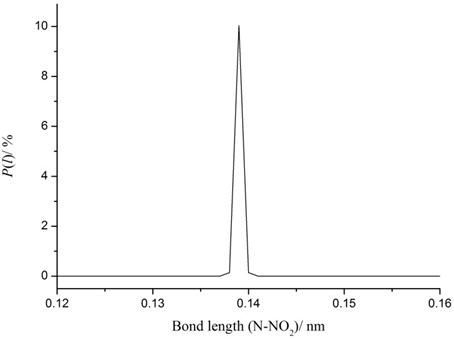Figure 7. Trigger bond length distribution.

From Fig. 7, we can see that the trigger bond length distribution is like symmetric Gauss’s distribution and more than 95% of the trigger bond has length of 0.133~0.144 nm. From Table 3, we can see that the probable trigger bond length (Lprob) and average trigger bond length (Lave) are nearly the same and both of them don’t vary obviously, while the maximum trigger bond length (Lmax) decreases monotonously with the increasing of absorbed number of water. The decreasing of maximum trigger bond length means that it may be less likely for the trigger bond to be broken when subjected to external stimulus, thus indicating that the sensitivity of composition B decrease after adsorption. In other words, adsorption water has positive effect on sensitivity of explosive and the safety of composition B can be improved after adsorption.

Table 3. Trigger bond length (nm).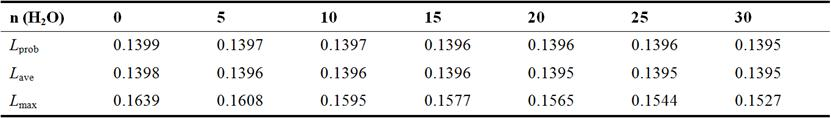3.5.2. Interaction Energy of Trigger Bond

The interaction energy of trigger bond (EN—N) is defined as follows: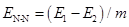(7)

Where E1 is the total energy of the system after reaching equilibrium state, E2 represents the total equilibrium energy of the system with all the N atoms in RDX fixed purposely, m refers the number of N—NO2 bond in composition B. EN—N can be used to measure the bond strength and judge the safety of energetic materials. The higher the value of EN—N is, the stronger the bond strength is.

Take the (1 0 0) crystal surface as an example, Fig. 8 presents the interaction energy of trigger bond (EN—N) in different conditions.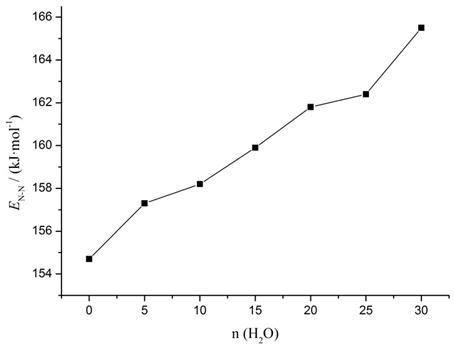Figure 8. Interaction energy of trigger bond.

What can be concluded from Fig. 8 is that with the increasing of absorbed number of water, the interaction energy of trigger bond increases gradually. Before adsorption, the interaction energy of trigger bond is 154.7 kJ/mol, after adsorption, the energy is 165.5 kJ/mol, the increasing of interaction energy of trigger bond is 6.98%. The increasing of the interaction energy of trigger bond means that the strength of the N—NO2 bond becomes stronger and it becomes hard for the trigger bond to be broken to cause decomposition or explosion for the energetic materials. That is to say, the sensitivity of composition B decreases after adsorption.

3.5.3. Cohesive Energy Density

Cohesive energy density (CED) is defined as the energy that needed for a material to transfer from condensed phase to gas phase. Cohesive energy density equals to van der Waals plus with the electrostatic. Table 4 presents the cohesive energy density and other energies of the equilibrium system.

What can be drawn from Table 4 is that with the increasing of adsorbed number of water, the cohesive energy density, van der Waals and electrostatic increases. After adsorption, the increasing extent of cohesive energy density, van der Waals and electrostatic is 29.75%, 46.27%, 19.86%, respectively. The increasing of cohesive energy density implies that the sensitivity of explosive decreases, which is consistent with the conclusions drawn above.

Table 4. CED and other energies (kJ/cm3)c).

 n (H2O) 0 5 10 15 20 25 30 Cohesive Energy Density 0.679 0.724 0.745 0.783 0.831 0.852 0.881 Van Der Waals 0.255 0.279 0.297 0.317 0.349 0.356 0.373 Electrostatic 0.423 0.445 0.448 0.467 0.482 0.496 0.507

c) Cohesive Energy Density = Van Der Waals + Electrostatic.

4. Conclusions

This paper mainly researches the adsorption mechanism of water on composition B crystal surfaces and the effect on mechanical properties and sensitivity of explosive by molecular dynamics simulation method. The results show that:

(1) After adsorption water, the mechanical properties of composition B decreases, which illustrates that the mechanical properties becomes worse, so the adsorption effect on mechanical properties should be taken into consideration and some correlated measures should be taken to ensure that the explosive can keep its favorable mechanical properties.

(2) The (0 1 0) crystal surfaces has the best adsorption capacity, then comes to the (1 0 0) crystal surfaces, and (0 0 1) is the last. For all of the three crystal surfaces, with the increasing of adsorbed number of water, the adsorption energy decreases, thus illustrating that the adsorption capacity increases.

(3) After adsorption, the maximum trigger bond length of explosive decreases, while the interaction energy of trigger bond and cohesive energy density increases, which indicates that the sensitivity of composition B decreases and the safety has been improved. Therefore, the adsorption has advantages on the safety of explosive.

References

1. Xi Peng, Nan Hai, Ni Bing, Yang Jiangang, Jia Xianzhen, Zheng Yafeng, "Vulnerability of composition B by bullet penetration," Chin. J. Energ. Mater, vol. 22, pp. 62-65, 2014.
2. Ren Xiaoning, Chang Hai, Shao Yinghui, Wang Keyong, Wang Hongxing, "Aging behavior of modified composition B explosive charge," Chin. J. Explos. Propellants, vol. 36, pp. 37-41, 2013.
3. Discover Accelrys. Material Studio 3.0, San Diego, CA, 2004.
4. Zhao Li, Xiao Jijun, Chen Jun, Ji Guangfu, Zhu Wei, Zhao Feng, Wu Qiang, Xiao Heming, "Molecular dynamics study on the relationships of modeling, structural structure and energy properties with sensitivity for RDX-based PBXs," Sci. Sinica Chem, vol. 43, pp. 576-584, 2013.
5. Xiao Jijun, Zhao Li, Zhu Wei, Chen Jun, Ji Guangfu, Zhao Feng, Wu Qiang, Xiao Heming, "Molecular dynamics study on the relationships of modeling, structural and energy properties with sensitivity for RDX-based PBXs," Sci. China Chem, vol. 55, pp. 2587-2594, 2012.
6. Xiao Jijun, Li Songyuan, Chen Jun, Ji Guangfu, Zhu Wei, Zhao Feng, Wu Qiang, Xiao Heming, "Molecular dynamics study on the correlation between structure and sensitivity for defective RDX crystals and their PBXs," J. Mol. Model, vol. 19, pp. 803-809, 2013.
7. Sun Ting, Liu Qiang, Xiao Jijun, Zhao Feng, Xiao Heming, "Molecular dynamics simulation of interface interactions and mechanical properties of CL-20/HMX cocrystal and its based PBXs," Acta Chim. Sinica, vol. 72, pp. 1036-1042, 2014.
8. Sun Ting, Xiao Jijun, Zhao Feng, Xiao Heming, "Molecular dynamics simulation of compatibility, interface interactions and mechanical properties of CL-20/DNB cocrystal based PBXs," Chin. J. Energ. Mater, vol. 23, pp. 309-314, 2015.
9. Zhang Xiang, Wang Yuling, "Molecular dynamics simulation of adsorption of mixed gases on JOB-9003 surfaces," Chin. J. Explos. Propellants, vol. 37, pp. 48-52, 2014.
10. Xu Xiaojuan, Xiao Jijun, Huang Hui, Li Jinshan, Xiao Heming, "Molecular dynamic simulations on the structures and properties of ε-CL-20 (0 0 1)/F2314 PBX," J. Hazard. Mater, vol. 175, pp. 423-428, 2010.
11. Wang Yuling, Guo yanan, "Effects of surface adsorption on mechanical properties of JO-9159 explosive by molecular dynamics simulation," Chin. J. Explos. Propellants, vol. 39, pp. 80-85, 2016.
12. Zhu Wei, Xiao Jijun, Zhu Weihua, Xiao Heming, "Molecular dynamics simulations of RDX and RDX-based plastic-bonded explosives," J. Hazard. Mater, vol. 164, pp. 1082-1088, 2009.
13. Xiao Jijun, Wang Wenrui, Chen Jun, Ji Guangfu, Zhu Wei, Xiao Heming, "Study on the relations of sensitivity with energy properties for HMX and HMX-based PBXs by molecular dynamics simulation," Physica B, vol. 407, pp. 3504-3509, 2012.
14. J. H. Weiner, Statistical Mechanics of Elasticity, John Wiley, New York, 1983.
15. Hooks DE, Ramos KJ, Bolme CA, Cawkwell MJ, "Elasticity of crystalline molecular explosives," Propellants, Explos., Pyrotech, vol. 40, pp. 333-350, 2015.
16. S. F. Pugh, "Relation between the elastic moduli and the plastic properties of polycrystalline pure metals," Philos. Mag. A, vol. 45 pp. 823-843, 1954.
17. Sun H, "Compass: An ab initio force-field optimized for condense-phase applications- overview with details on alkanes and benzene compounds," J. Phys. Chem. B, vol. 102, pp. 7338-7364, 1998.
18. Sun H, Ren P, Fried J R, "The COMPASS Force Field: Parameterization and validation for phosphazenes," Comput. Theore. Polym. Sci, vol. 8, pp. 229-246, 1998.
19. Bunte S W, Sun H, "Molecular modeling of energetic materials: the parameterization and validation of nitrate esters in the COMPASS Forcefield," J. Chem. Chem. B, vol. 104, pp. 2477-2489, 2000.
20. Bowden F P, Yoffe A D, Initiation and growth of explosion in liquids and solids, Cambridge: Cambridge University Press, 1952.
21. Kamlet M J, Adoiph H G, "The relationship of impact sensitivity with structure of organic high explosives," Propellants, Explos., Pyrotech, vol. 4, pp. 30-34, 1979.
22. Mullay J, "Relationship between impact sensitivity and molecular electronic structure," Propellants, Explos., Pyrotech, vol. 12, pp. 121-124, 1987.
23. Owens F J, Jayasuriya K, Abrahmsen L, "Computational analysis of some properties associated with the nitro groups in polynitroaromatic molecules," Chem. Phys. Lett, vol. 116, pp. 434-438, 1985.
24. Xiao Heming, Wang Zunyao, Yao Jianmin, "Quantum chemical study on sensitivity and stability of aromatic nitro explosives I: nitro derivatives of amino-benzenes," Acta Chim. Sinica, vol. 43, pp. 14-18, 1985.
25. Turner A G, Davis L P, "Thermal decomposition of TNT: use of 1-nitropropene to model the initial stages of decomposition," J. Am. Chem. Soc, vol. 106, pp. 5447-5451, 1984.

 Contents 1. 2. 2.1. 2.2. 2.3. 2.4. 3. 3.1. 3.2. 3.3. 3.4. 3.5. 4.
Article ToolsAbstractPDF(1122K)